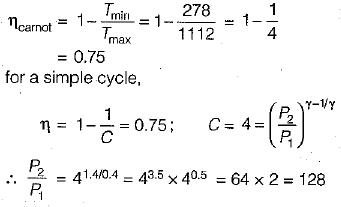Courses

# Gas Turbine Power Plants - 2

## 10 Questions MCQ Test Topicwise Question Bank for GATE Mechanical Engineering | Gas Turbine Power Plants - 2

Description
This mock test of Gas Turbine Power Plants - 2 for Mechanical Engineering helps you for every Mechanical Engineering entrance exam. This contains 10 Multiple Choice Questions for Mechanical Engineering Gas Turbine Power Plants - 2 (mcq) to study with solutions a complete question bank. The solved questions answers in this Gas Turbine Power Plants - 2 quiz give you a good mix of easy questions and tough questions. Mechanical Engineering students definitely take this Gas Turbine Power Plants - 2 exercise for a better result in the exam. You can find other Gas Turbine Power Plants - 2 extra questions, long questions & short questions for Mechanical Engineering on EduRev as well by searching above.
QUESTION: 1

### Consider the following statements: 1. The speed of rotation of the moving elements of gas turbines is much higher than of steam turbine. 2. Gas turbine plants are heavier and larger in size than steam power plants. 3. Gas turbines require cooling water for their operations. 4. Any kind of fuel can be used with gas turbines. Which of the statements given above are correct?

Solution:

Specific weight of gas turbine plant is smaller than steam power plant, so it is used in aircraft. Cooling water in gas turbine is used for intercooling purpose.

QUESTION: 2

### Consider the following statements about gas turbine: 1. The maximum temperature is limited to about 1000 to 1200 K. 2. Rotational speed of turbine blades limits the maximum temperature. 3. Blades are generally made of nickel-cobalt alloy. 4. The blade tip is hottest part. Which of the above statements are correct?

Solution:

(i) Metallurgical limit of turbine blade limits the maximum temperature in a gas turbine cycle.
(ii) The turbine blade has uniform temperature throughout its entire height.

QUESTION: 3

### The combustion efficiency of a gas turbine using good combustor is of the order of

Solution:
QUESTION: 4

The A/F ratio for a gas turbine is generally kept closer to

Solution:
QUESTION: 5

Which of the following statement is false pertaining to a constant pressure gas turbine?

Solution:
QUESTION: 6

The mechanical losses are accounted on

Solution:
QUESTION: 7

The value of dynamic temperature for air flow having velocity of 100 m/s at atmospheric temperature is about

Solution:

The stagnation enthalpy h0 is the enthalpy which a gas stream of enthalpy h and velocity v would possess when brought to rest adiabatically and without work transfer.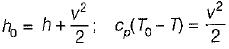∴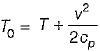T is static temperature and v2/2cp is dynamic temperature.
Dynamic temperature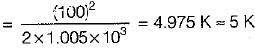QUESTION: 8

In a gas turbine cycle, the turbine output is 800 kj/kg, the compressor work is 500 kj/kg and the heat supplied is 1200 kj/kg. The thermal efficiency of the cycle is

Solution:

Network = WT- WC
= 800 - 500 = 300 kJ/kg
Heat supplied = 1200 kJ/kg
Thermal efficiency = Network/Heat applied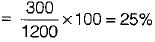QUESTION: 9

An open cycle constant pressure gas turbine uses a fuel of calorific value 45000 kJ/kg with A/F ratio 60 : 1 and develops a net output of 100 kj/kg of air. The thermal efficiency of the cycle is

Solution: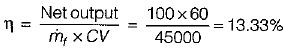QUESTION: 10

A gas turbine operates between 5°C and 839°C. What is the pressure ratio at which cycle efficiency equals carnot cycle efficiency?

Solution: